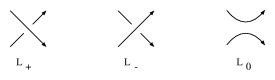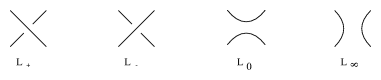Difference between revisions of "Conway skein triple"

Three oriented link diagrams, or tangle diagrams, $L_+$, $L_-$, $L_0$ in $\mathbf R^3$, or more generally, in any three-dimensional manifold, that are the same outside a small ball and in the ball look likeFigure: c130240a

Similarly one can define the Kauffman bracket skein triple of non-oriented diagrams $L_+$, $L_0$ and $L_\infty$, and the Kauffman skein quadruple, $L_+$, $L_-$, $L_0$ and $L_\infty$, used to define the Brandt–Lickorish–Millett–Ho polynomial and the Kauffman polynomial:Figure: c130240b

Generally, a skein set is composed of a finite number of $k$-tangles and can be used to build link invariants and skein modules (cf. also Skein module).

How to Cite This Entry:
Conway skein triple. Encyclopedia of Mathematics. URL: http://encyclopediaofmath.org/index.php?title=Conway_skein_triple&oldid=14693
This article was adapted from an original article by Jozef Przytycki (originator), which appeared in Encyclopedia of Mathematics - ISBN 1402006098. See original article